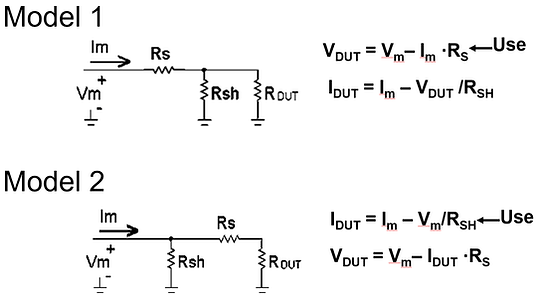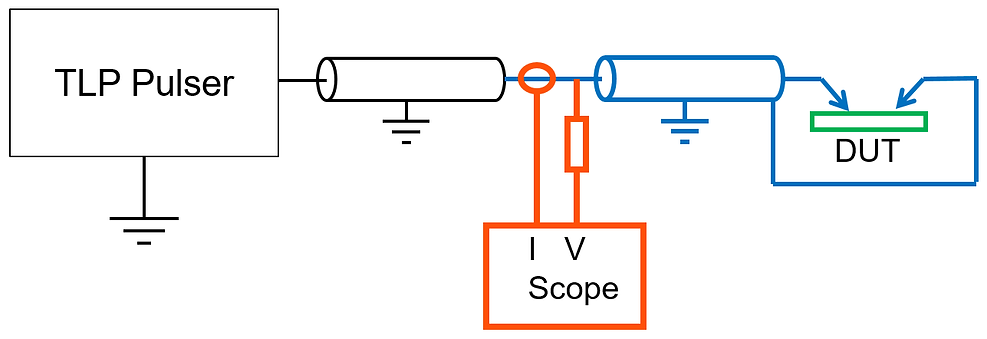top of page

## TLP Calibration

Like most measurement techniques, TLP needs calibration for TLP measurements made with different equipment or at different locations or times to provide consistent results.  TLP system data processing needs to apply corrections to the raw measurement data taken from oscilloscope recordings pulses so that the data points plotted in a TLP system pulsed I-V curve output properly report the correct voltages and currents.  This can be thought of a mapping from raw measured data to calibrated (correct or true) results.

Calibration is a term that is often used to include verification and corrections.  We need to make a destination between these three terms.  Verification is the measurement of known standards to check that tester operation is within expected limits.  Corrections are adjustments made to the TLP raw data so that known distortions of the measurements are removed.  Calibration, in the formal sense, is the process of determining the relation between the output of a measuring instrument and the value of the input quantity (a known device to be used as a measurement standard), and then adjusting the output from the measurement instrument to agree with known value of the standard device.

Verification is done by measuring known standard device(s) and comparing the measurement output with the known values. Passing the verification means finding the measured value is within a predetermined tolerance, such as 5 or 10%, of the known true value.  Common standard devices are often contained in a package or assembly called an “SOLZ,” which stands for Short, Open, Load, and Zener.  The load is a resistor, and values between 5 and 50 ohms are good for TLP operation verification as device resistances in this region are common.  The Zener diode is measured in its reverse breakdown as a voltage reference to verify voltage measurements.  Zener diodes with voltages close to 6 V typically have the lowest thermal coefficients (TC) and hence are the most stable.  To verify higher voltages with low TC, several diodes can be connected in series.Figure 1:  Uncorrected TLP Data

Corrections are usually made using the Short and Open of the SOLZ, or testing with other ways of measuring references of 0 Ω and ∞ Ω.   Measuring these “reference devices” on an uncorrected TLP system (so the raw data is plotted on the I-V curve) would typically result in the data shown in Fig 1.  The uncorrected measurement of a short usually will measure to be a few ohms, which can be attributed to the resistance of the cable between the voltage measurement probe and the device under test (DUT) as well as contact resistances at the DUT.  A short should produce data points on the vertical (current) axis as the voltage across a short is always zero.  A correction is needed to move (rotate) the raw data short circuit points to approximately lie on the current axis.  Similarly, the open circuit points (current is always zero) should all lie approximately on the horizontal (voltage) axis.  Current probe response and oscilloscope offsets may contribute to the open circuit measurement error.  Fig. 2 shows the highlighted section of a TLP system that corrections address.Figure 2: Components in blue dealt with by the correction

The Correction can be modelled as removing the errors from a series resistance, Rs, and shunt resistance, Rsh, that lies between the measurement probes location and the DUT.  As shown in Fig. 3, there can be two possible lumped circuit models constructed depending where Rsh is located relative to Rs.  As the resistances being modeled are truly distributed and not lumped, neither model is exact.  One model may be used for current correction and the other for voltage correction, and the use of two can be justified as they are equivalent when Rs << Rsh.Figure 3:  circuit models of correction needed

Correction parameters can be determined by measuring Rs and Rsh at a selected TLP pulse voltage using

Measure Rsh = Vm/Im with open DUT,

Measure Rs = Vm/Im with shorted DUT, and

then finding the corrected V DUT and IDUT from the raw measured data VM and IM for all subsequent measurements with the equations in Fig. 3.

Corrections for the open and short circuit responses should be done periodically and when any component from the oscilloscope to the DUT is changed.  Since DUT impedances during the TLP pulse are often low, the short circuit correction is the most critical.

A calibration can be conducted after the open/short corrections have been made.  While the corrections can be thought as rotating the I-V points around the origin to align open and short measurements with the axes, calibration can be thought as stretching the axes so the voltage points and current points are properly scaled, as in Fig 4.  Calibration is adjusting the scaling of the current and voltage probes and the oscilloscope.  Calibration focuses on the TLP system components highlighted in Fig. 5.  A voltage source and a current source would be ideal reference devices for calibration, but they don’t actually exist.  A Zener diode is a practical approximation to a voltage source.  The Zener voltage can be determined, by measuring it with a Source-Meter Unit (SMU) that forces a preset current through the diode.  The SMU itself should be calibrated and traceable to national standard such as NIST.  Calibration implies traceability; the definition from NIST is, “Traceability requires the establishment of an unbroken chain of comparisons to stated references each with a stated uncertainty.”  The Zener should be measured at the same current with TLP as the diode doesn’t has an ideal zero on resistance.  Care should taken not use too much current to prevent i2R heating during measurement that could change the diodes temperature significantly.Figure 4:  Correction rotation and Calibration stretching data pointsFigure 5:  Components in red dealt with by the calibration

There is not a simple component that approximates a current source for calibrating the TLP current measurement.  However, once the voltage has been calibrated using a Zener voltage reference, the current can be calibrated using a resistor, the Load in SOLZ.  Trusting the voltage calibration and using a known resistor, the current scale can be adjusted by a calibration multiplier to have the TLP output properly report the resistor.

The mathematics of Calibration is more straight forward than that of Corrections as simple multiplying factors can be used.  By using known “transfer standard devices” the calibration factors can be found from TLP measurements using

Measure KV = VKNOWN / VM with known Zener, and

Measure KI = (VDUT / RKNOWN) / IDUT with known Resistor,

Then calibration factors are applied by VDUT = KV • VM, and IDUT = KI • IM.

A new Calibration should be made at least annually, or after a change in equipment setup or whenever the verification fails.

Combining both corrections and calibration factors the raw measured values, VM and IM, of voltage and current can be mapped to the reportable DUT measurements VDUT and IDUT using linear algebra.  Making the voltage and currents vectors and by multiplying the raw measured value vector by the matrix of corrections/calibrations to obtain the calibrated values vector usingbottom of page